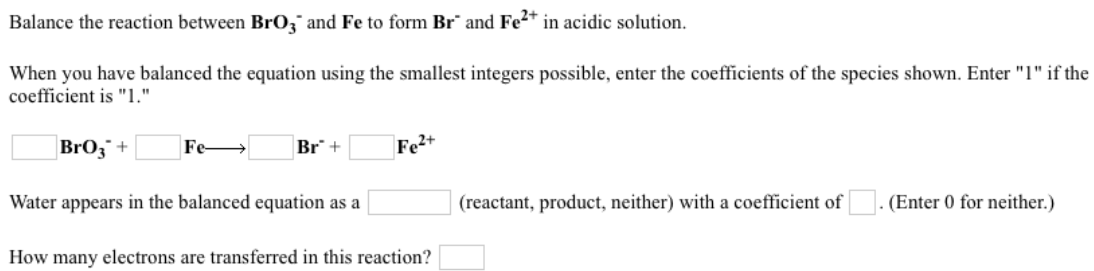# Balance the reaction between BrO3^- and Fe to form Br^- and Fe^2+ in acidic solution. When you have balanced the equation using the smallest integers possible, enter the coefficients of the species shown. Enter "l" if the coefficient is "l". ___ BrO3^- + ___ Fe → ___ Br^- + ___ Fe^2+ Water appears in the balanced equation as ____ (reactant, product, neither) with a coefficient of ___. (Enter 0 for neither.) How many electrons are transferred in this reaction?